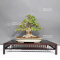##### The Japanese Bonsai specialist
Direct order Contact Help / Services Newsletter# Acer buergerianum 16080231

Acer buergerianumref. : 12010

2.340,00

Available quantity : 1Order

###### Description

Exceptional bonsaï for passionate collectors.

It comes from Tomoya Nishikawa's nursery in Himeji.

Over 40 years old, this bonsaï presents an impressive trunk height of 285 mm and a branch spread of approximately 330 mm.

It was repotted in March 2023 in a handcrafted Chinese enameled signed pottery, from Yixing, with dimensions of 360*260*45 mm.

Moyogi style with beautifully developed roots.

The massive trunk measures about 90-100 mm in diameter, with an exceptional nebari of 190-200 mm.

The bark is exquisitely formed, offering a harmonious trunk conicity.

The branches are carefully shaped and ramified, without cutting wounds or wire marks, demonstrating meticulous attention to the care of this tree.

This bonsai was photographed in April 2023 on a display stand, which is not included.

It is a rare collector's piece, specially selected by Guy Maillot, for passionate bonsai lovers. Have you considered purchasing this book?

#bonsai 6.6 #this 4.4 #trunk 3.5 #with 3.4 #buergerianum 3.2 #exceptional 3.1 #passionate 3 #unique 2.6 #from 2.4 #acer 2.4

Formule
(( ROUND((CHAR_LENGTH(b.article_nom)-CHAR_LENGTH(REPLACE(b.article_nom, 'bonsai', '')))/LENGTH('bonsai')) + ROUND((CHAR_LENGTH(b.article_description)-CHAR_LENGTH(REPLACE(b.article_description, 'bonsai', '')))/LENGTH('bonsai')) ) * 4.6) + (( ROUND((CHAR_LENGTH(b.article_nom)-CHAR_LENGTH(REPLACE(b.article_nom, 'this', '')))/LENGTH('this')) + ROUND((CHAR_LENGTH(b.article_description)-CHAR_LENGTH(REPLACE(b.article_description, 'this', '')))/LENGTH('this')) ) * 4.4) + (( ROUND((CHAR_LENGTH(b.article_nom)-CHAR_LENGTH(REPLACE(b.article_nom, 'trunk', '')))/LENGTH('trunk')) + ROUND((CHAR_LENGTH(b.article_description)-CHAR_LENGTH(REPLACE(b.article_description, 'trunk', '')))/LENGTH('trunk')) ) * 3.5) + (( ROUND((CHAR_LENGTH(b.article_nom)-CHAR_LENGTH(REPLACE(b.article_nom, 'with', '')))/LENGTH('with')) + ROUND((CHAR_LENGTH(b.article_description)-CHAR_LENGTH(REPLACE(b.article_description, 'with', '')))/LENGTH('with')) ) * 3.4) + (( ROUND((CHAR_LENGTH(b.article_nom)-CHAR_LENGTH(REPLACE(b.article_nom, 'buergerianum', '')))/LENGTH('buergerianum')) + ROUND((CHAR_LENGTH(b.article_description)-CHAR_LENGTH(REPLACE(b.article_description, 'buergerianum', '')))/LENGTH('buergerianum')) ) * 3.2) + (( ROUND((CHAR_LENGTH(b.article_nom)-CHAR_LENGTH(REPLACE(b.article_nom, 'exceptional', '')))/LENGTH('exceptional')) + ROUND((CHAR_LENGTH(b.article_description)-CHAR_LENGTH(REPLACE(b.article_description, 'exceptional', '')))/LENGTH('exceptional')) ) * 3.1) + (( ROUND((CHAR_LENGTH(b.article_nom)-CHAR_LENGTH(REPLACE(b.article_nom, 'passionate', '')))/LENGTH('passionate')) + ROUND((CHAR_LENGTH(b.article_description)-CHAR_LENGTH(REPLACE(b.article_description, 'passionate', '')))/LENGTH('passionate')) ) * 3) + (( ROUND((CHAR_LENGTH(b.article_nom)-CHAR_LENGTH(REPLACE(b.article_nom, 'acer', '')))/LENGTH('acer')) + ROUND((CHAR_LENGTH(b.article_description)-CHAR_LENGTH(REPLACE(b.article_description, 'acer', '')))/LENGTH('acer')) ) * 2.4) + (( ROUND((CHAR_LENGTH(b.article_nom)-CHAR_LENGTH(REPLACE(b.article_nom, 'from', '')))/LENGTH('from')) + ROUND((CHAR_LENGTH(b.article_description)-CHAR_LENGTH(REPLACE(b.article_description, 'from', '')))/LENGTH('from')) ) * 2.4) + (( ROUND((CHAR_LENGTH(b.article_nom)-CHAR_LENGTH(REPLACE(b.article_nom, 'demonstrating', '')))/LENGTH('demonstrating')) + ROUND((CHAR_LENGTH(b.article_description)-CHAR_LENGTH(REPLACE(b.article_description, 'demonstrating', '')))/LENGTH('demonstrating')) ) * 2.3)

## Secure payment## Delivery

Our logistic partners :04 74 55 23 48
Pépinière MAILLOT-BONSAÏ
Le Bois Frazy
01990 RELEVANT - FRANCE
on appointment